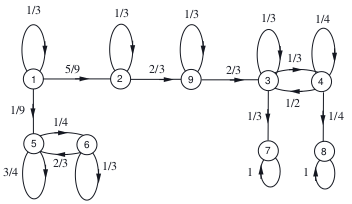# generate multiple Vars and vectors with these Vars

Hi

this exercise comes from a MOOC course that has just ended EDX.org , MITx: 6.431x Probability - The Science of Uncertainty and Dataforget()
var('pi_1 pi_2 pi_3 pi_4 pi_5 pi_6 pi_7 pi_8 pi_9')
# matrix of the Markov's Chain
m = matrix([   \
[1/3 , 5/9 ,  0  ,  0  , 1/9 ,  0  ,  0  ,  0  ,  0   ], \
[ 0  , 1/3 ,  0  ,  0  ,  0  ,  0  ,  0  ,  0  , 2/3  ], \
[ 0  ,  0  , 1/3 , 1/3 ,  0  ,  0  , 1/3 ,  0  ,  0   ], \
[ 0  ,  0  , 1/2 , 1/4 ,  0  ,  0  ,  0  , 1/4 ,  0   ], \
[ 0  ,  0  ,  0  ,  0  , 3/4 , 1/4 ,  0  ,  0  ,  0   ], \
[ 0  ,  0  ,  0  ,  0  , 2/3 , 1/3 ,  0  ,  0  ,  0   ], \
[ 0  ,  0  ,  0  ,  0  ,  0  ,  0  ,  1  ,  0  ,  0   ], \
[ 0  ,  0  ,  0  ,  0  ,  0  ,  0  ,  0  ,  1  ,  0   ], \
[ 0  ,  0  , 2/3 ,  0  ,  0  ,  0  ,  0  ,  0  , 1/3  ]  \

])
v=vector([pi_1,pi_2,pi_3,pi_4,pi_5,pi_6,pi_7,pi_8,pi_9])
#Assuming that the Markov chain is initially in state 2 (i.e., X0=2),
# what is the probability that the chain eventually reaches state 7?
#################################
# brute force
#x_0=2
v_s=vector([0,1,0,0,0,0,0,0,0])
#v_s=vector([0,0,1,0,0,0,0,0,0])

V_r=v_s*m^110
show(V_r.n())
#################################
# smart way
eqT=[]
for i in range(0,len(v)):
eqT.append(m[i]*v==v[i])
show(eqT)
show(eqT)
S=solve([eqT,eqT,pi_7==1,pi_8==0],pi_3,pi_4,pi_7,pi_8)
show(S)
show("probability that the chain eventually reaches state 7 : ",S)


I would like to generate the variables, and the vector automaticaly: kind of:

dimM=m.dimensions()
varStr=''
for i in range(0,dimM):
#varStr=varStr+ ' a_'+str(i+1)
varStr=varStr+ ' a_'+str(i)

show(varStr)
var(varStr)
R = PolynomialRing(QQ, dimM, 'a_')
v= vector(R,dimM, R.gens())
show(m)
show(v)
eqT=[]
for i in range(0,len(v)):
show(m[i]*v==v[i])
eqT.append(m[i]*v==v[i])
#show(eqT)


but unfortunately this code just above for generating variables and vector automaticaly does not work

edit retag close merge delete

Sort by » oldest newest most votedI can't run the code right now (and you didn't include the output / the actual problem). The problem is with generating the equations, yes? The point is that equality testing in the polynomial ring R will give a yes or no answer, instead of the symbolic (unevaluated) equality that you want. To get the symbolic equality, convert to the symbolic ring SR beforehand. I guess it would be SR(m[i]*v) == SR(v[i]). Probably you can also avoid using the symbolic ring, but that is a separate story.

more

which code does not work ?, for me the first code is ok and gives the good anwser. the second code is about generating n variables (a_i or pi_i ) i :1 to n and vector with theses variables.

in order to write only the matrix of size n x n, and to generate the variables and the vector automatically.

the question of the problem is in the first code:

thank you rburing it is ok using SR():

for i in range(0,len(v)): eqT.append(SR(m[i]*v)==SR(v[i])) show(eqT)

how I can say you give the answer,(because you write your answer in the comment part of the post